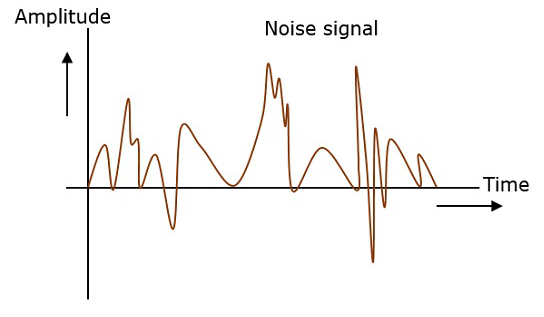# Principles of Communication - Noise

In any communication system, during the transmission of the signal, or while receiving the signal, some unwanted signal gets introduced into the communication, making it unpleasant for the receiver, questioning the quality of the communication. Such a disturbance is called as Noise.

## What is Noise?

Noise is an unwanted signal which interferes with the original message signal and corrupts the parameters of the message signal. This alteration in the communication process, leads to the message getting altered. It is most likely to be entered at the channel or the receiver.

The noise signal can be understood by taking a look at the following example.Hence, it is understood that noise is some signal which has no pattern and no constant frequency or amplitude. It is quite random and unpredictable. Measures are usually taken to reduce it, though it can’t be completely eliminated.

Most common examples of noise are −

• Buzz sound amidst of telephone conversations

• Flicker in television receivers, etc.

## Effects of Noise

Noise is an inconvenient feature which affects the system performance. Following are the effects of noise.

### Noise limits the operating range of the systems

Noise indirectly places a limit on the weakest signal that can be amplified by an amplifier. The oscillator in the mixer circuit may limit its frequency because of noise. A system’s operation depends on the operation of its circuits. Noise limits the smallest signal that a receiver is capable of processing.

### Noise affects the sensitivity of receivers

Sensitivity is the minimum amount of input signal necessary to obtain the specified quality output. Noise affects the sensitivity of a receiver system, which eventually affects the output.

## Types of Noise

The classification of noise is done depending on the type of the source, the effect it shows or the relation it has with the receiver, etc.

There are two main ways in which noise is produced. One is through some external source while the other is created by an internal source, within the receiver section.

### External Source

This noise is produced by the external sources which may occur in the medium or channel of communication, usually. This noise cannot be completely eliminated. The best way is to avoid the noise from affecting the signal.

### Examples

Most common examples of this type of noise are −

• Atmospheric noise (due to irregularities in the atmosphere).

• Extra-terrestrial noise, such as solar noise and cosmic noise.

• Industrial noise.

### Internal Source

This noise is produced by the receiver components while functioning. The components in the circuits, due to continuous functioning, may produce few types of noise. This noise is quantifiable. A proper receiver design may lower the effect of this internal noise.

### Examples

Most common examples of this type of noise are −

• Thermal agitation noise (Johnson noise or Electrical noise).

• Shot noise (due to the random movement of electrons and holes).

• Transit-time noise (during transition).

• Miscellaneous noise is another type of noise which includes flicker, resistance effect and mixer generated noise, etc.

## Signal to Noise Ratio

Signal-to-Noise Ratio (SNR) is the ratio of the signal power to the noise power. The higher the value of SNR, the greater will be the quality of the received output.

Signal-to-noise ratio at different points can be calculated by using the following formulae −

$$Input \: SNR = (SNR)_I = \frac{Average \: power \: of \: modulating \: signal}{Average \: power \: of \: noise \: at \: input}$$

$$Output \: SNR = (SNR)_O = \frac{Average \: power \: of \: demodulated \: signal}{Average \: power \: of \: noise \: at \: output}$$

$$Channel \: SNR = (SNR)_C = \frac{Average \: power \: of \: modulated \: signal}{Average \: power \: of \: noise \: in \: message \: bandwidth}$$

## Figure of Merit

The ratio of output SNR to the input SNR can be termed as the Figure of merit (F). It is denoted by F. It describes the performance of a device.

$$F = \frac{(SNR)_O}{(SNR)_I}$$

Figure of merit of a receiver is −

$$F = \frac{(SNR)_O}{(SNR)_C}$$

It is so because for a receiver, the channel is the input.